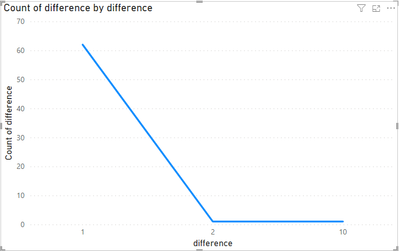cancel
Showing results for
Did you mean:Helper I

## Count of value for a Measure

Is it even possible to get the count for the DAX measure below?

Delta T100 - T0 =
CALCULATE(MIN(T0_T100_Delta_Distibution[value]) , T0_T100_Delta_Distibution[Folder1] = "t100")
-
CALCULATE(MIN(T0_T100_Delta_Distibution[value]) , T0_T100_Delta_Distibution[Folder1] = "t0")

I've been trying for weeks on my own.

First, I need a measure to show each unique value for the Delta column, Delta T100-T0.
Second, I need to show the count of occurrences for each unique value.

The expected table and visual must be derived from the table contained in the pbix.  Attached at the bottom.
Expected Table

 Delta T100-T0: Unique Values Count of Unique Values 1 62 2 1 10 1

Expected Visual   ( I mocked this visual)1 ACCEPTED SOLUTIONSolution Specialist

Hey Ron,

See changed pbix here. Maybe there's a different way but I essentially created a summary table of your data (change it or add columns as needed) so I could use the deltas from that summary table as the min and max values of another table (a parameter table). That parameter table was used to calculate your counts.

----------------------------------
If this post helps, please consider accepting it as the solution to help other members find it quickly. Also, don't forget to hit that thumbs up and subscribe! (Oh, uh, wrong platform?)

5 REPLIES 5Helper I

Hi  @Wilson_

In practice, T0 represents data before testing begins.  Subsequent Tn data (T100, T125, T150), will represent data after hours of conditioning.  Tn = 100 hours, 125 hours, 150 hours.  The device is tested at each Tn interval.

I want to compare the delta from T0 vs T100 and T125 and T150.  Then see the frequency distribution of the deltas between T100 vs T125 vs T150 for every single delta value.

The real data table will be over 50K rows, and as much as 500K rows.  Depending on the sample size.

The expected deltas are expected to change as the conditioning hours lengthen.
When the delta frquency distribitions are compared in a single visual we should be able to see that trend.Solution Specialist

Hello Ron,

I'm happy to help.

However, I'm struggling to figure out what you're trying to accomplish, even looking at the pbix and your expected visual. Can you maybe rephrase and/or use a smaller table of data to illustrate your expected results? What is the Delta T100-T0 measure supposed to calculate, in words? The measure itself doesn't make sense to me.Helper I

@Wilson_
In the screen capture below, I've highlighted, in green, three different measures that calculate the same thing.
Which is the difference between column t100 and t0.

I'm trying to get the count of occurances for each unique value in the delta columns.  Highlighted in green
In other words, I'm trying to get the count of the measure.  For each and every delta value in the column.  Just one of the delta measures.  Not all the delta measures.

I've been trying everything: count, countx, counta.   Unsuccessfully.

I'm sure it can be done.  I'm just not that much of an expert at Power BI DAX to figure it out myself.Solution Specialist

Hey Ron,

See changed pbix here. Maybe there's a different way but I essentially created a summary table of your data (change it or add columns as needed) so I could use the deltas from that summary table as the min and max values of another table (a parameter table). That parameter table was used to calculate your counts.

----------------------------------
If this post helps, please consider accepting it as the solution to help other members find it quickly. Also, don't forget to hit that thumbs up and subscribe! (Oh, uh, wrong platform?)Helper I

@Wilson_

Thank you! I've been away from any PBI development that last month.  Now, it's due.  Oh boy.
I'll check it out.  Thanks for your time again....